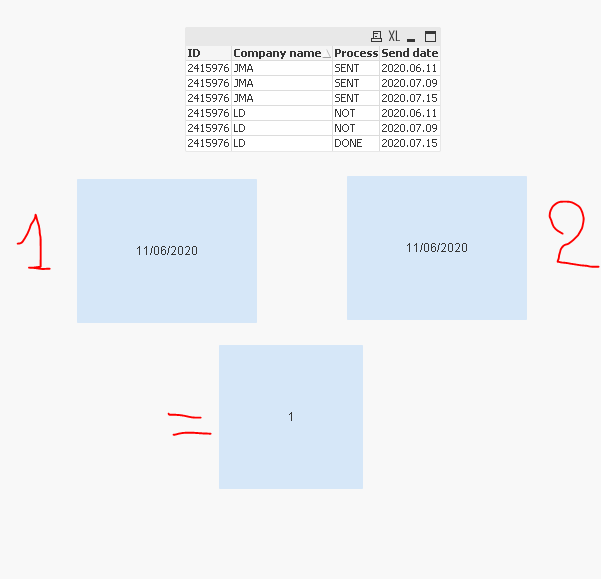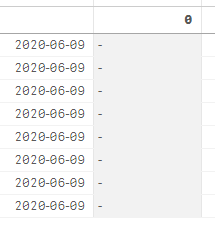# New to Qlik Sense

If you’re new to Qlik Sense, start with this Discussion Board and get up-to-speed quickly.

Announcements
Our May 2021 end-to-end product release from Data Integration to Data Analytics is out! READ DETAILS
cancel
Showing results for
Search instead for
Did you mean:Creator

## Counting days between two dates, but wrong answer, when the dates are the same day

Hello all,

Got a problem. I'm counting days between two dates, I need to count how many network days are between the lowest date and the second to the lowest, grouped by ID.

The example:

 ID Company name Process Send date 2415976 JMA SENT 2020.06.11 2415976 LD NOT 2020.06.11 2415976 JMA SENT 2020.07.09 2415976 LD NOT 2020.07.09 2415976 JMA SENT 2020.07.10 2415976 LD DONE 2020.07.10

My measure expression looks like this:

avg(networkdays(aggr(min([Send date]),[ID]),aggr(min([Send date],2),[ID])))

Now, somehow it skips the second date, which is equal to the 1st one '2020.06.11', and brings the result 21, which is between '2020.07.09' and '2020.06.11'

The outcome should look like this:

 ID NetWorkDaysBetween 2415976 0

Thanks for the help!

2 Solutions

Accepted SolutionsMVP

@Edvin  one change I did is to removed total. Does it gives correct at that level? What would be the output if you expand the second

avg(aggr(NetWorkDays(min(total <[Bylos/Subbylos numeris]>{<DateFlag={1}>}[Siuntimo data]),
min(total <[Bylos/Subbylos numeris]>{<DateFlag={2}>}[Siuntimo data])), [Bylos/Subbylos numeris]))MVP

@Edvin Updated expression

``````Avg(Aggr(
NetWorkDays(
FirstSortedValue(DISTINCT [Siuntimo data], Aggr([Siuntimo data]+Rank(TOTAL [Serviso pavadinimas])/1e10, [Bylos/Subbylos numeris], [Serviso pavadinimas], [Siuntimo data])),
FirstSortedValue(DISTINCT [Siuntimo data], Aggr([Siuntimo data]+Rank(TOTAL [Serviso pavadinimas])/1e10, [Bylos/Subbylos numeris], [Serviso pavadinimas], [Siuntimo data]), 2)
),
[Bylos/Subbylos numeris]))``````

Also, noticed that same date within NetWorkDays() function gives 1. If you want one less day, then do -1 at the end

20 RepliesMaster II

Hi @Edvin ,

1-NetWorkDaysBetween two date

if two dates are equal Networkday will return 1 if it's a working day otherwise 0.

Example :

=networkdays ('30/09/2020', '30/09/2020') ->1

=networkdays ('03/10/2020', '03/10/2020')->0

2- for the problem I propose you another alternative

for the first min use :

``````=Date(textbetween(concat(
aggr(nodistinct min([Send date]),ID,[Send date]),','),'',','))``````

and for the second min use :

``````=Date(textbetween(concat(
aggr(nodistinct min([Send date]),ID,[Send date]),','),',',','))``````

So the networdday script wil be :

``````=networkdays(
Date(textbetween(concat(
aggr(nodistinct min([Send date]),ID,[Send date]),','),'',',')),
Date(textbetween(concat(
aggr(nodistinct min([Send date]),ID,[Send date]),','),',',','))
)``````

I tested for this example :and also for this :Regards,
Taoufiq ZARRA

"Please LIKE posts and "Accept as Solution" if the provided solution is helpful "

(you can mark up to 3 "solutions") 😉MVP

@Edvin  I would recommend to create Flag in script to make your expression simple and easy to understand. Also it will be good as performance point of view.

``````Data:
LOAD ID,
[Company name],
Process,
date(Date#([Send date],'YYYY.MM.DD')) as [Send date]
FROM
[Book2.xlsx]
(ooxml, embedded labels, table is Sheet1);

Final:
NoConcatenate
LOAD *,
if(ID<>Peek(ID),1,RangeSum(Peek(DateFlag),1)) as DateFlag
Resident Data
Order by ID,[Send date];

DROP Table Data;``````

Now you can simply use below expression

``````=NetWorkDays(Only({<DateFlag={1}>}[Send date]),Only({<DateFlag={2}>}[Send date]))-1

or

=NetWorkDays(Only({<DateFlag={1}>}[Send date]),Only({<DateFlag={2}>}[Send date]))``````Creator
Author

@Taoufiq_Zarra  Thanks for the help, but that doesn't work. If two dates are equal and it's a working day, it doesn't give me the result 1, that's the problem. And your alternative solution doesn't work too.

@KushSomething's wrong. I used the 1st expression, and it only shows for the TOTAL line the correct answer..Also, I got lots of ID number's so I need it averaged, but when I do it with aggr(avg(EXPRESSION),[ID]) it doesn't bring anything.MVP

@Edvin  what is the dimensions you are using.Creator
Author

I've got [ID], [Event date], [First sent date], [Company city], [Company name], [Send date],[Process].

Not sure what it will give you.

Maybe it is better to attach .qvf file?MVP

@Edvin  If you can attach Qvf that will be helpful. try below meanwhile f you are following the approach I suggested

``=avg(total <ID>aggr(NetWorkDays(Only(total <ID>{<DateFlag={1}>}[Send date]),Only(total <ID>{<DateFlag={2}>}[Send date])), ID))``MVP

@Edvin May be try this

``````NetWorkDays(
FirstSortedValue([Send date], Aggr([Send date]+Rank([Company name])/1e10, ID, [Company name], [Send date])),
FirstSortedValue([Send date], Aggr([Send date]+Rank([Company name])/1e10, ID, [Company name], [Send date]), 2)
)``````Creator
Author

@Kushthanks! It works, but not as intended fully.

I've got one more problem, for every ID there is a responsible USER, for which I'm trying to calculate this expression. How much days (on average) do we get the 1st response (network days between those two dates).The last column is the one I did with your expression, it's the same value for everyone.

Gosh... It's so complicated to just calculate networkdays between two dates :D.

If it's impossible to help, tell me, I will attach .qvf fileMVP

@Edvin  I would advise to attach qvf